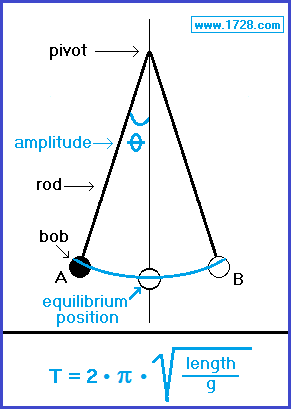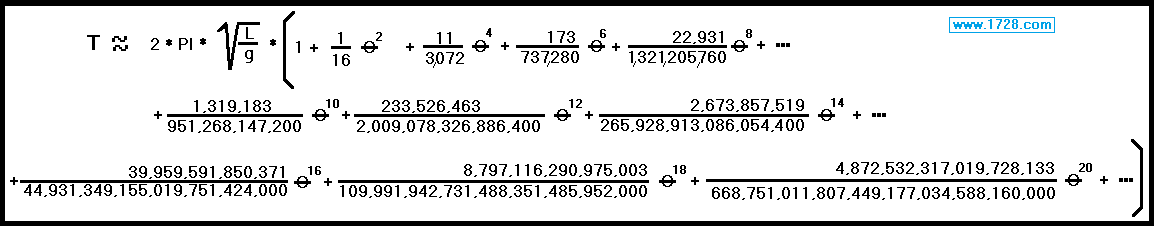Pendulum Calculator

 Will you be inputing meters or feet ? Radians or Degrees? Input pendulum length >>>>> Input pendulum amplitude >>> Input "g" (default 9.80665)>>> The box below is not for input. By Formula   + Theta2   + Theta4   + Theta6   + Theta8   + Theta10   + Theta12   + Theta14     Total * * *    Using AGM     This calculator displays the pendulum period (in seconds) for various values of theta. The last line "AGM" (Arithmetic-Geometric Mean) shows the exact value.A pendulum is a bob (or weight), suspended from a pivot by a rod, so that it may swing freely back and forth when it is displaced from its equilibrium position (or low point). The period of a pendulum is the time it takes for it to travel from point A to point B and return to point A. The formula for a pendulum's period is: 2 • π √  len / g   where the period is in seconds, length is in meters and "g" is the gravitational acceleration at the surface of the Earth which has a standard value of 9.80665 meters per second2. This formula works well provided that the pendulum's angle from the perpidicular (amplitude) is no greater than 10 degrees, but when it becomes greater than 10 degrees, the formula becomes much more complex:The above formula can be used to the extent of just how much accuracy you want. Most sources suggest using the formula up to θ6. Fortunately, an exact formula has been discovered for a pendulum's period and it involves taking the arithmetic geometric mean Click here for the exact formula.

Significant Figures >>>
 For easy readability, the answers are displayed in a "significant figure" format which "neatens up" answers such as 77.3333333333333333. Numbers larger than 1,000 will be displayed in scientific notation (and the same number of significant figures). You may change the significant figures displayed by changing the number in the box above. Internet Explorer and most other browsers, will display the answers properly but there are a few browsers that will show no output whatsoever. If so, enter a zero in the box above. This eliminates all formatting but it is better than seeing no output at all.